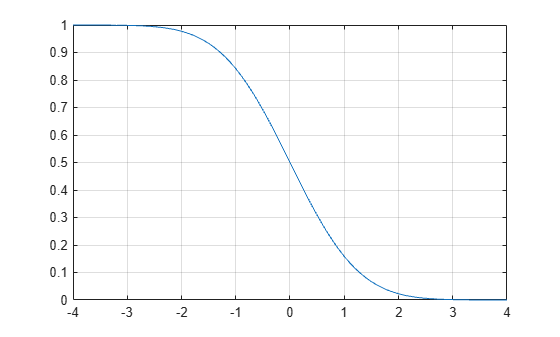# qfunc

## Syntax

``y = qfunc(x)``

## Description

example

````y = qfunc(x)` returns the output of the Q function for each element of the real-valued input. The Q function is (1 – f), where f is the result of the cumulative distribution function of the standardized normal random variable. For more information, see Algorithms.```

## Examples

collapse all

Calculate the Q function values for a real-valued input vector.

```x = -4:0.1:4; y = qfunc(x);```

Plot the results.

```plot(x,y) grid```Calculate the QPSK error probability at an ${\mathit{E}}_{\mathrm{b}}/{\mathit{N}}_{0}$ setting of 7 dB by using the Q function.

Convert the ${\mathit{E}}_{\mathrm{b}}/{\mathit{N}}_{0}$ in dB to its linear equivalent.

```ebnodB = 7; ebno = 10^(ebnodB/10);```

Using the Q function, calculate the QPSK error probability, ${P}_{b}=Q\left(\sqrt{2\frac{{E}_{b}}{{N}_{0}}}\right)$.

`Pb = qfunc(sqrt(2*ebno))`
```Pb = 7.7267e-04 ```

## Input Arguments

collapse all

Input, specified as a real-valued scalar, matrix, or array.

Data Types: `double`

## Output Arguments

collapse all

Q function output, returned as a scalar, matrix, or array. `y` has the same dimensions as input `x`. Output values are in the range [0, 1].

## Algorithms

For a scalar x, the Q function is (1 – f), where f is the result of the cumulative distribution function of the standardized normal random variable. The Q function is defined as

`$Q\left(x\right)=\frac{1}{\sqrt{2\pi }}\underset{x}{\overset{\infty }{\int }}\mathrm{exp}\left(-{t}^{2}/2\right)dt$`

The Q function is related to the complementary error function, erfc, according to

`$Q\left(x\right)=\frac{1}{2}\text{erfc}\left(\frac{x}{\sqrt{2}}\right)$`

## Version History

Introduced before R2006a